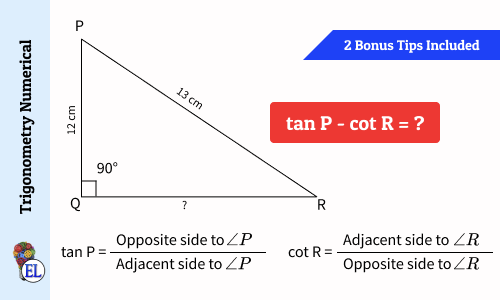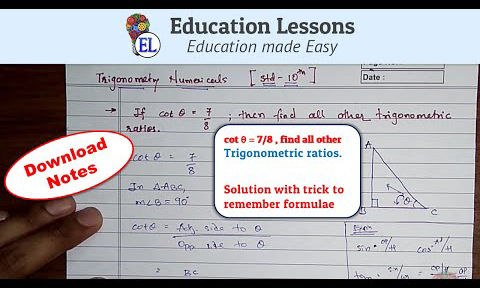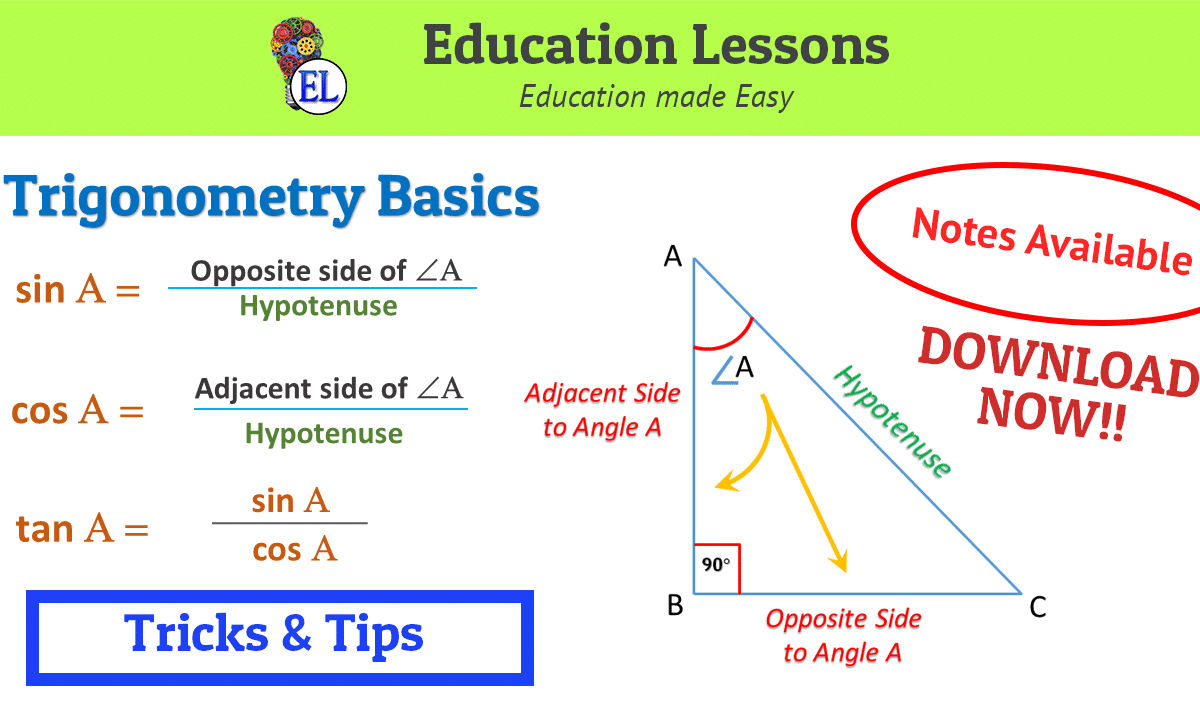Imp. Questions Trigonometry

View all
tan (A + B) = √3 and tan (A -B) = 1/√3 | Trigonometry Numerical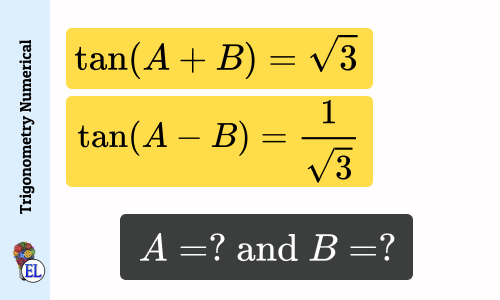Includes bonus question
15 cot A = 8 | Find the value of sin A and sec A | Trigonometry Numerical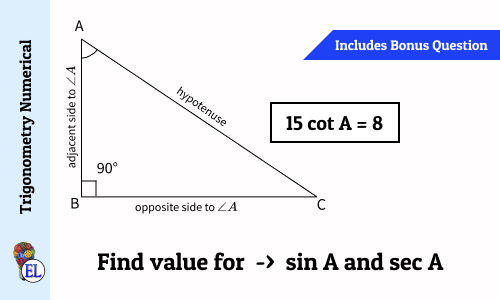2 bonus tips
PQ = 12cm and PR = 13cm | Find tan P - cot R | Trigonometry Numerical